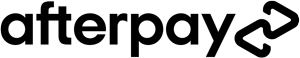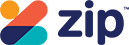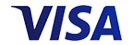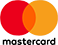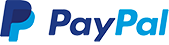Grid List
WAS \$39.95 NOW \$29.96
Increase value Decrease value
WAS \$59.95 NOW \$44.96
Increase value Decrease value
WAS \$44.95 NOW \$37.46
Increase value Decrease value
WAS \$49.95 NOW \$37.46
Increase value Decrease value
WAS \$49.95 NOW \$37.46
Increase value Decrease value
WAS \$21.95 NOW \$16.46
Increase value Decrease value
WAS \$21.95 NOW \$16.46
Increase value Decrease value
WAS \$19.95 NOW \$14.96
Increase value Decrease value
WAS \$19.95 NOW \$14.96
Increase value Decrease value
WAS \$14.95 NOW \$11.21
Increase value Decrease value
\$12.95
Increase value Decrease value
\$29.95
Increase value Decrease value